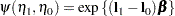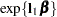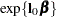### Odds or Odds Ratio

The odds ratio is the exponentiation of a difference on the logit scale,andandare the corresponding odds. If the ODDSRATIO option is specified in a suitable model in the PROC GLIMMIX statement or the individual statements that support the option, odds ratios are computed in the "Odds Ratio Estimates" table (MODEL statement), the "Differences of Least Squares Means" table (LSMEANS / DIFF), and the "Simple Effect Comparisons of Least Squares Means" table (LSMEANS / SLICEDIFF=). Odds are computed in the "Least Squares Means" table.